Need help? Email help@shout.education

# pH Curves (Titration Curves)

## The Equivalence Point of a Titration

### Sorting Out Some Confusing Terms

When you carry out a simple acid-base titration, you use an indicator to tell you when you have the acid and alkali mixed in exactly the right proportions to "neutralise" each other. When the indicator changes colour, this is often described as the end point of the titration.

In an ideal world, the colour change would happen when you mix the two solutions together in exactly equation proportions. That particular mixture is known as the equivalence point.

For example, if you were titrating sodium hydroxide solution with hydrochloric acid, both with a concentration of 1 mol dm-3, 25 cm3 of sodium hydroxide solution would need exactly the same volume of the acid – because they react 1 : 1 according to the equation.

\text{NaOH}_{(aq)} + \text{HCl}_{(aq)} \longrightarrow \text{NaCl}_{(aq)} + \text{H}_2\text{O}_{(l)}

In this particular instance, this would also be the neutral point of the titration, because sodium chloride solution has a pH of 7.

But that isn't necessarily true of all the salts you might get formed.

For example, if you titrate ammonia solution with hydrochloric acid, you would get ammonium chloride formed. The ammonium ion is slightly acidic, and so pure ammonium chloride has a slightly acidic pH.

That means that at the equivalence point (where you had mixed the solutions in the correct proportions according to the equation), the solution wouldn't actually be neutral. To use the term "neutral point" in this context would be misleading.

Similarly, if you titrate sodium hydroxide solution with ethanoic acid, at the equivalence point the pure sodium ethanoate formed has a slightly alkaline pH because the ethanoate ion is slightly basic.

• The term "neutral point" is best avoided.
• The term "equivalence point" means that the solutions have been mixed in exactly the right proportions according to the equation.
• The term "end point" is where the indicator changes colour. As you will see on the page about indicators, that isn't necessarily exactly the same as the equivalence point.

Note: You can find out about indicators by following this link (also available from the acid-base equilibria menu). You should read the present page first though.

## Simple pH curves

All the following titration curves are based on both acid and alkali having a concentration of 1 mol dm-3. In each case, you start with 25 cm3 of one of the solutions in the flask, and the other one in a burette.

Although you normally run the acid from a burette into the alkali in a flask, you may need to know about the titration curve for adding it the other way around as well. Alternative versions of the curves have been described in most cases.

### Titration Curves for Strong Acid vs. Strong Base

We'll take hydrochloric acid and sodium hydroxide as typical of a strong acid and a strong base.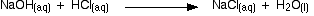#### Running acid into the alkali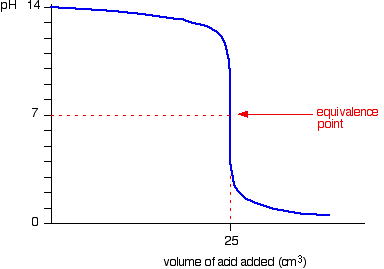You can see that the pH only falls a very small amount until quite near the equivalence point. Then there is a really steep plunge. If you calculate the values, the pH falls all the way from 11.3 when you have added 24.9 cm3 to 2.7 when you have added 25.1 cm3.

Note: If you need to know how to calculate pH changes during a titration, you may be interested in my chemistry calculations book.

#### Running alkali into the acid

This is very similar to the previous curve except, of course, that the pH starts off low and increases as you add more sodium hydroxide solution.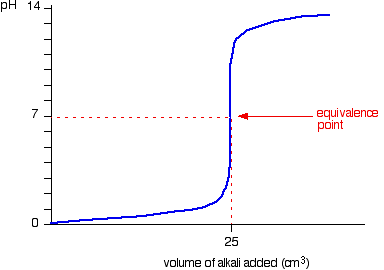Again, the pH doesn't change very much until you get close to the equivalence point. Then it surges upwards very steeply.

### Titration Curves for Strong Acid vs. Weak Base

This time we are going to use hydrochloric acid as the strong acid and ammonia solution as the weak base.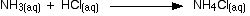#### Running acid into the alkali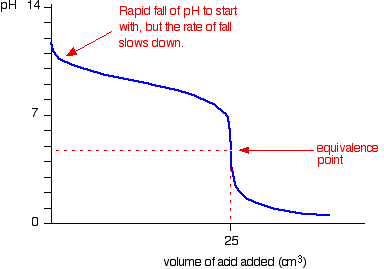Because you have got a weak base, the beginning of the curve is obviously going to be different. However, once you have got an excess of acid, the curve is essentially the same as before.

At the very beginning of the curve, the pH starts by falling quite quickly as the acid is added, but the curve very soon gets less steep. This is because a buffer solution is being set up – composed of the excess ammonia and the ammonium chloride being formed.

Note: You can find out more about buffer solutions by following this link. However, this is a very minor point in the present context, and you would probably do better to read the whole of the current page before you follow this up.

Notice that the equivalence point is now somewhat acidic ( a bit less than pH 5), because pure ammonium chloride isn't neutral. However, the equivalence point still falls on the steepest bit of the curve. That will turn out to be important in choosing a suitable indicator for the titration.

#### Running alkali into the acid

At the beginning of this titration, you have an excess of hydrochloric acid. The shape of the curve will be the same as when you had an excess of acid at the start of a titration running sodium hydroxide solution into the acid.

It is only after the equivalence point that things become different.

A buffer solution is formed containing excess ammonia and ammonium chloride. This resists any large increase in pH – not that you would expect a very large increase anyway, because ammonia is only a weak base.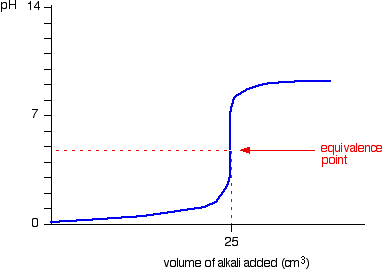### Titration Curves for Weak Acid vs. Strong Base

We'll take ethanoic acid and sodium hydroxide as typical of a weak acid and a strong base.

\text{CH}_3\text{COOH}_{(aq)} + \text{NaOH}_{(aq)} \longrightarrow \text{CH}_3\text{COONa}_{(aq)} + \text{H}_2\text{O}_{(l)}

#### Running acid into the alkali

For the first part of the graph, you have an excess of sodium hydroxide. The curve will be exactly the same as when you add hydrochloric acid to sodium hydroxide. Once the acid is in excess, there will be a difference.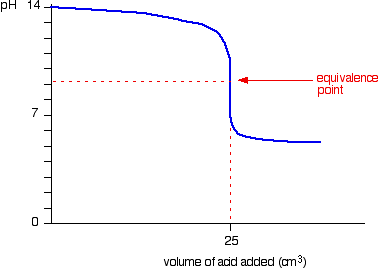Past the equivalence point you have a buffer solution containing sodium ethanoate and ethanoic acid. This resists any large fall in pH.

#### Running alkali into the acid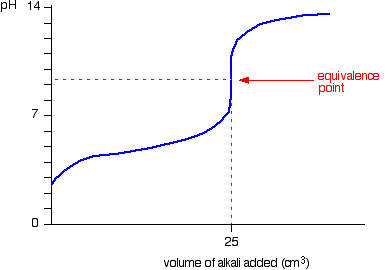The start of the graph shows a relatively rapid rise in pH but this slows down as a buffer solution containing ethanoic acid and sodium ethanoate is produced. Beyond the equivalence point (when the sodium hydroxide is in excess) the curve is just the same as that end of the HCl – NaOH graph.

### Titration Curves for Weak Acid vs. Weak Base

The common example of this would be ethanoic acid and ammonia.

\text{CH}_3\text{COOH}_{(aq)} + \text{NH}_{3(aq)} \longrightarrow \text{CH}_3\text{COONH}_{4(aq)}

It so happens that these two are both about equally weak – in that case, the equivalence point is approximately pH 7.

#### Running acid into the alkali

This is really just a combination of graphs you have already seen. Up to the equivalence point it is similar to the ammonia – HCl case. After the equivalence point it is like the end of the ethanoic acid – NaOH curve.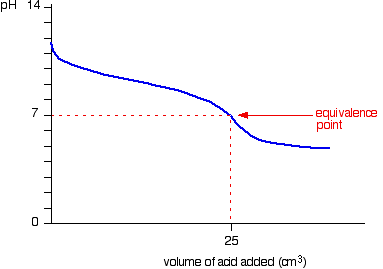Notice that there isn't any steep bit on this graph. Instead, there is just what is known as a "point of inflexion". That lack of a steep bit means that it is difficult to do a titration of a weak acid against a weak base.

Note: Because you almost never do titrations with this combination, there is no real point in giving the graph where they are added the other way round. It isn't difficult to work out what it might look like if you are interested – take the beginning of the sodium hydroxide added to ethanoic acid curve, and the end of the ammonia added to hydrochloric acid one.

The reason that it is difficult to do these titrations is discussed on the page about indicators.

The way you normally carry out a titration involves adding the acid to the alkali. Here are reduced versions of the graphs described above so that you can see them all together.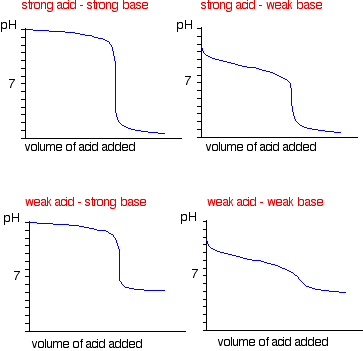## More Complicated Titration Curves

### Adding Hydrochloric acid to Sodium carbonate Solution

The overall equation for the reaction between sodium carbonate solution and dilute hydrochloric acid is:

\text{Na}_2\text{CO}_{3(aq)} + 2\text{HCl}_{(aq)} \longrightarrow 2\text{NaCl}_{(aq)} + \text{CO}_{2(g)} + \text{H}_2\text{O}_{(l)}

If you had the two solutions of the same concentration, you would have to use twice the volume of hydrochloric acid to reach the equivalence point – because of the 1 : 2 ratio in the equation.

Suppose you start with 25 cm3 of sodium carbonate solution, and that both solutions have the same concentration of 1 mol dm-3. That means that you would expect the steep drop in the titration curve to come after you had added 50 cm3 of acid.

The actual graph looks like this: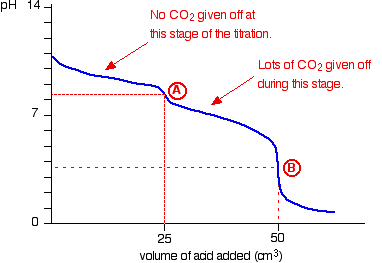The graph is more complicated than you might think – and curious things happen during the titration.

You expect carbonates to produce carbon dioxide when you add acids to them, but in the early stages of this titration, no carbon dioxide is given off at all.

Then – as soon as you get past the half-way point in the titration – lots of carbon dioxide is suddenly released.

The graph is showing two end points – one at a pH of 8.3 (little more than a point of inflexion), and a second at about pH 3.7. The reaction is obviously happening in two distinct parts.

In the first part, complete at A in the diagram, the sodium carbonate is reacting with the acid to produce sodium hydrogencarbonate:

\text{Na}_2\text{CO}_{3(aq)} + \text{HCl}_{(aq)} \longrightarrow \text{NaCl}_{(aq)} + \text{NaHCO}_{3(aq)}

You can see that the reaction doesn't produce any carbon dioxide.

In the second part, the sodium hydrogencarbonate produced goes on to react with more acid – giving off lots of CO2.

\text{NaHCO}_{3(aq)} + \text{HCl}_{(aq)} \longrightarrow \text{NaCl}_{(aq)} + \text{CO}_{2(g)} + \text{H}_2\text{O}_{(l)}

That reaction is finished at B on the graph.

It is possible to pick up both of these end points by careful choice of indicator. That is explained on the separate page on indicators.

### Adding Sodium hydroxide Solution to Dilute Ethanedioic acid

Ethanedioic acid was previously known as oxalic acid. It is a diprotic acid, which means that it can give away 2 protons (hydrogen ions) to a base. Something which can only give away one (like HCl) is known as a monoprotic acid.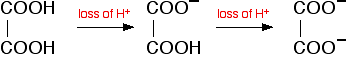The reaction with sodium hydroxide takes place in two stages because one of the hydrogens is easier to remove than the other. The two successive reactions are: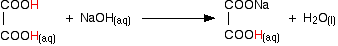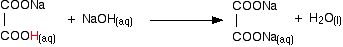If you run sodium hydroxide solution into ethanedioic acid solution, the pH curve shows the end points for both of these reactions.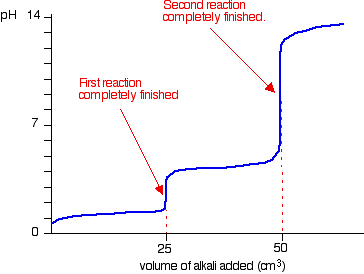The curve is for the reaction between sodium hydroxide and ethanedioic acid solutions of equal concentrations.